The Australian Journal of Mathematical Analysis and Applications

 Home News Editors Volumes RGMIA Subscriptions Authors Contact

ISSN 1449-5910

You searched for zhou
Total of 77 results found in site

8: Paper Source PDF document

Paper's Title:

On Subspace-Supercyclic Operators

Author(s):

Mansooreh Moosapoor

Assistant Professor,
Department of Mathematics,
Farhangian University, Tehran,
Iran.
E-mail: mosapor110@gmail.com m.mosapour@cfu.ac.ir

Abstract:

In this paper, we prove that supercyclic operators are subspace-supercyclic and by this we give a positive answer to a question posed in ( L. Zhang, Z. H. Zhou, Notes about subspace-supercyclic operators, Ann. Funct. Anal., 6 (2015), pp. 60--68). We give examples of subspace-supercyclic operators that are not subspace-hypercyclic. We state that if T is an invertible supercyclic operator then Tn and T-n is subspace-supercyclic for any positive integer n. We give two subspace-supercyclicity criteria. Surprisingly, we show that subspace-supercyclic operators exist on finite-dimensional spaces.

5: Paper Source PDF document

Paper's Title:

Uniform Convergence of Schwarz Method for Noncoercive Variational Inequalities Simple Proof

Author(s):

Department of mathematics, LANOS Laboratory,
Faculty of the Sciences, University Badji Mokhtar,
P.O 23000 Annaba,
Algeria.

haiourm@yahoo.fr,

Abstract:

In this paper we study noncoercive variational inequalities, using the Schwarz method. The main idea of this method consists in decomposing the domain in two subdomains. We give a simple proof for the main result concerning L error estimates, using the Zhou geometrical convergence and the L approximation given for finite element methods by Courty-Dumont.

5: Paper Source PDF document

Paper's Title:

L∞- Error Estimate of Schwarz Algorithm for Elliptic Quasi-Variational Inequalities Related to Impulse Control Problem

Author(s):

Lab. LANOS, Department of Mathematics,
P.O.Box 12, Annaba 23000,
Algeria.

Lab. LAIG, Department of Mathematics,
University May 8th 1945,
P.O.Box 401, Guelma 24000,
Algeria.

allmehri@yahoo.fr

Abstract:

In this work, we study Schwarz method for a class of elliptic quasi-variational inequalities. The principal result of this investigation is to prove the error estimate in ∞-norm for two domains with overlapping nonmatching grids, using the geometrical convergence, and the uniform convergence of Cortey Dumont.

4: Paper Source PDF document

Paper's Title:

A Low Order Least-Squares Nonconforming Finite Element Method for Steady Magnetohydrodynamic Equations

Author(s):

Z. Yu, D. Shi and H. Zhu

College of Science,
Zhongyuan University of Technology,
Zhengzhou 450007,
China.
E-mail: 5772@zut.edu.cn

School of Mathematics and Statistics,
Zhengzhou University,
Zhengzhou 450001,
China.
E-mail: shi_dy@126.com

Mathematics Department,
University of Southern Mississippi,
Hattiesburg MS, 39406,
U.S.A
E-mail: huiqing.zhu@usm.edu

Abstract:

A low order least-squares nonconforming finite element (NFE) method is proposed for magnetohydrodynamic equations with EQ1rot element and zero-order Raviart-Thomas element. Based on the above element's typical interpolations properties, the existence and uniqueness of the approximate solutions are proved and the optimal order error estimates for the corresponding variables are derived.

3: Paper Source PDF document

Paper's Title:

On a Hilbert-type Inequality with the Polygamma Function

Author(s):

Bing He and Bicheng Yang
Department of Mathematics, Guangdong Education College,
Guangzhou, 510303,
China.

Department of Mathematics, Guangdong Education College,
Guangzhou, 510303,
China.

Abstract:

By applying the method of weight function and the technique of real analysis, a Hilbert-type inequality with a best constant factor is established, where the best constant factor is made of the polygamma function. Furthermore, the inverse form is given.

2: Paper Source PDF document

Paper's Title:

On an Extension of Hilbert’s Integral Inequality with Some Parameters

Author(s):

Bicheng Yang

Department of Mathematics, Guangdong Education College, Guangzhou, Guangdong 510303, People’s Republic of China.
URL
:

Abstract:

In this paper, by introducing some parameters and estimating the weight function, we give an extension of Hilbert’s integral inequality with a best constant factor. As applications, we consider the equivalent form and some particular results.

2: Paper Source PDF document

Paper's Title:

Compactly Supported Interpolatory Orthogonal Multiwavelet Packets

Author(s):

Yang Shouzhi

Department of Mathematics,
Shantou University,
Shantou, Po Box 515063,
P.R.China.
szyang@stu.edu.cn

Abstract:

Compactly supported interpolatory orthogonal multiwavelet packets are introduced. Precisely, if both the multiscaling function and the corresponding multiwavelet have the same interpolatory property, then the multiwavelet packets are also interpolatory orthogonal. Thus, the coefficients of decomposition or synthesis of multiwavelet packets can be realized by sampling instead of inner products. This multiwavelet packets provide a finer decomposition of multiwavelet packets space and give a better localization.

2: Paper Source PDF document

Paper's Title:

Oscillations of First Order Linear Delay Difference Equations

Author(s):

G. E. Chatzarakis and I. P. Stavroulakis

Department of Mathematics, University of Ioannina,
451 10, Greece
ipstav@cc.uoi.gr

Abstract:

Consider the first order linear delay difference equation of the form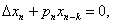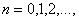where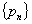is a sequence of nonnegative real numbers, k is a positive integer and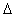denotes the forward difference operator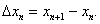New oscillation criteria are established when the well-known oscillation conditions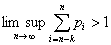and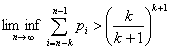are not satisfied. The results obtained essentially improve known results in the literature.

2: Paper Source PDF document

Paper's Title:

A Strengthened Hardy-Hilbert's Type Inequality

Author(s):

Weihong Wang and Bicheng Yang

Department of Mathematics, Guangdong Education Institute,
Guangzhou, Guangdong 520303,
People's Republic Of China
wwh@gdei.edu.cn
bcyang@pub.guangzhou.gd.cn
URL: http://www1.gdei.edu.cn/yangbicheng/index.html

Abstract:

By using the improved Euler-Maclaurin's summation formula and estimating the weight coefficient, we give a new strengthened version of the more accurate Hardy-Hilbert's type inequality. As applications, a strengthened version of the equivalent form is considered.

2: Paper Source PDF document

Paper's Title:

Necessary and Sufficient Conditions for Uniform Convergence and Boundedness of a General Class of Sine Series

Author(s):

Laszlo Leindler

Bolyai Institute, University of Szeged,
értanúk tere 1,
H-6720 Szeged,
Hungary.
leindler@math.u-szeged.hu

Abstract:

For all we know theorems pertaining to sine series with coefficients from the class γGBVS give only sufficient conditions. Therefore we define a subclass of γGBVS in order to produce necessary and sufficient conditions for the uniform convergence and boundedness if the coefficients of the sine series belong to this subclass; and prove two theorems of this type.

2: Paper Source PDF document

Paper's Title:

Existence Results for Perturbed Fractional Differential Inclusions

Author(s):

Y.-K. Chang

Department of Mathematics,
Lanzhou Jiaotong University, Lanzhou, Gansu 730070, People's
Republic of China
lzchangyk@163.com

Abstract:

This paper is mainly concerned with the following fractional differential inclusions with boundary condition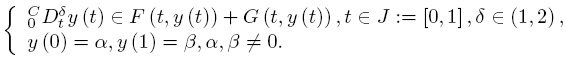A sufficient condition is established for the existence of solutions of the above problem by using a fixed point theorem for multivalued maps due to Dhage. Our result is proved under the mixed generalized Lipschitz and Carathéodory conditions.

2: Paper Source PDF document

Paper's Title:

Fekete-Szegö Problem for Univalent Functions with Respect to k-Symmetric Points

Author(s):

K. Al-Shaqsi and M. Darus

School of Mathematical Sciences, Faculty of Science and Technology,
University Kebangsaan Malaysia,
Bangi 43600 Selangor D. Ehsan,
Malaysia
ommath@hotmail.com
maslina@ukm.my

Abstract:

In the present investigation, sharp upper bounds of |a3- μa22| for functions f(z) = z + a2z2 + a2z3 + ... belonging to certain subclasses of starlike and convex functions with respect to k-symmetric points are obtained. Also certain applications of the main results for subclasses of functions defined by convolution with a normalized analytic function are given. In particular, Fekete- Szegö inequalities for certain classes of functions defined through fractional derivatives are obtained.

2: Paper Source PDF document

Paper's Title:

Nontrivial Solutions of Singular Superlinear Three-point Boundary Value Problems at Resonance

Author(s):

Feng Wang, Fang Zhang

School of Mathematics and Physics,
Changzou University,
Changzhou, 213164,
China.
fengwang188@163.com

Abstract:

The singular superlinear second order three-point boundary value problems at resonance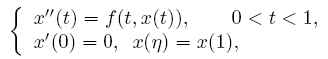are considered under some conditions concerning the first eigenvalues corresponding to the relevant linear operators, where n ∈ (0,1) is a constant, f is allowed to be singular at both t=0 and t=1. The existence results of nontrivial solutions are given by means of the topological degree theory.

2: Paper Source PDF document

Paper's Title:

Para-chaotic Tuples of Operators

Author(s):

Department of Mathematics,
Payame Noor University,
P.O. Box 19395-3697, Tehran,
Iran
b_yousefi@pnu.ac.ir

Abstract:

In this paper, we introduce para-chaotic tuples of operators and we give some relations between para-chaoticity and Hypercyclicity Criterion for a tuple of operators.

2: Paper Source PDF document

Paper's Title:

Hermite-Hadamard-Fejer Type Inequalities for Harmonically s-convex Functions via Fractional Integrals

Author(s):

İmdat İşcan, Mehmet Kunt

Department of Mathematics,
Faculty of Sciences and Arts,
Giresun University, Giresun,
Turkey.
E-mail: imdat.iscan@giresun.edu.tr

Department of Mathematics,
Faculty of Sciences,
61080, Trabzon,
Turkey.
E-mail: mkunt@ktu.edu.tr

Abstract:

In this paper, some Hermite-Hadamard-Fejer type integral inequalities for harmonically s-convex functions in fractional integral forms have been obtained.

2: Paper Source PDF document

Paper's Title:

On a Class of Meromorphic Functions of Janowski Type Related with a Convolution Operator

Author(s):

Abdul Rahman S. Juma, Husamaldin I. Dhayea

Department of Mathematics,
Iraq.
E-mail: dr_juma@hotmail.com

Department of Mathematics,
Tikrit University, Tikrit,
Iraq.

Abstract:

In this paper, we have introduced and studied new operator \$Qkλ,m,γ by the Hadamard product (or convolution) of two linear operators Dkλ and Im,γ, then using this operator to study and investigate a new subclass of meromorphic functions of Janowski type, giving the coefficient bounds, a sufficient condition for a function to belong to the considered class and also a convolution property. The results presented provide generalizations of results given in earlier works.

2: Paper Source PDF document

Paper's Title:

Ostrowski Type Inequalities for Lebesgue Integral: a Survey of Recent Results

Author(s):

Sever S. Dragomir1,2

1Mathematics, School of Engineering & Science
Victoria University, PO Box 14428
Melbourne City, MC 8001,
Australia
E-mail: sever.dragomir@vu.edu.au

2DST-NRF Centre of Excellence in the Mathematical and Statistical Sciences,
School of Computer Science & Applied Mathematics,
University of the Witwatersrand,
Private Bag 3, Johannesburg 2050,
South Africa
URL: http://rgmia.org/dragomir

Abstract:

The main aim of this survey is to present recent results concerning Ostrowski type inequalities for the Lebesgue integral of various classes of complex and real-valued functions. The survey is intended for use by both researchers in various fields of Classical and Modern Analysis and Mathematical Inequalities and their Applications, domains which have grown exponentially in the last decade, as well as by postgraduate students and scientists applying inequalities in their specific areas.

2: Paper Source PDF document

Paper's Title:

An Efficient Modification of Differential Transform Method for Solving Integral and Integro-differential Equations

Author(s):

S. Al-Ahmad, Ibrahim Mohammed Sulaiman*, and M. Mamat

Faculty of Informatics and Computing,
Universiti Sultan Zainal Abidin,
Terengganu, Besut Campus, 22200,
Malaysia.

Abstract:

In this paper, classes of integral and integro-differential equations are solved using a modified differential transform method. This proposed technique is based on differential transform method (DTM), Laplace transform (LT) procedure and Pad\'{e} approximants (PA). The proposed method which gives a good approximation for the true solution in a large region is referred to modified differential transform method (MDTM). An algorithm was developed to illustrate the flow of the proposed method. Some numerical problems are presented to check the applicability of the proposed scheme and the obtained results from the computations are compared with other existing methods to illustrates its efficiency. Numerical results have shown that the proposed MDTM method is promising compared to other existing methods for solving integral and integro-differential equations.

1: Paper Source PDF document

Paper's Title:

A Simple New Proof of Fan-Taussky-Todd Inequalities

Author(s):

Zhi-Hua Zhang and Zhen-Gang Xiao

Zixing Educational Research Section,
Chenzhou City, Hunan 423400, P. R. China.
Zhi-hua Zhang
Url: http://www.hnzxslzx.com/zzhweb/

Department Of Mathematics, Hunan Institute Of Science And Technology,
Yueyang City, Hunan 423400, P. R. China.
Zhen-gang Xiao

Abstract:

In this paper we present simple new proofs of the inequalities: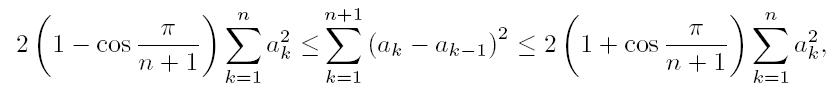which holds for all real numbers a0 = 0, a1, · · · , an, an+1 = 0 and the coefficients 2(1 - cos(π/(n + 1))) and 2(1 + cos(π/(n + 1))) are the best possible; and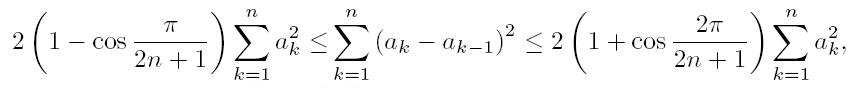which holds for all real numbers a0 = 0, a1, · · · , an and the coefficients 2(1-cos(π/(2n + 1))) and 2(1 + cos(π/(2n + 1))) are the best possible.

1: Paper Source PDF document

Paper's Title:

Logarithmically complete monotonicity properties for the gamma functions

Author(s):

Chao-Ping Chen and Feng Qi

Department of Applied Mathematics and Informatics,
Research Institute of Applied Mathematics,
Henan Polytechnic University,
Jiaozuo City, Henan 454010,
China.
chenchaoping@hpu.edu.cn

Department of Applied Mathematics and Informatics,
Research Institute of Applied Mathematics,
Henan Polytechnic University,
Jiaozuo City, Henan 454010,
China.
qifeng@hpu.edu.cn
fengqi618@member.ams.org

Abstract:

Some logarithmically completely monotonic functions involving the gamma functions are presented. As a consequence, some known results are proved and refined.

1: Paper Source PDF document

Paper's Title:

On Zeros of Diagonally Quasiconvex Multifunctions

Author(s):

Zoran D. Mitrović

Faculty of Electrical Engineering,
University of Banja Luka,
78000 Banja Luka, Patre 5
Bosnia and Herzegovina
zmitrovic@etfbl.net

Abstract:

In this paper, we extended the notion of diagonally quasiconvexity for multifunctions and established several existence results for zeros of diagonally quasiconvex multifunctions. As applications we obtain the results of fixed points, coincidence points and best approximations for multifunctions. Using our result we also prove the existence of solutions to the variational-like inequality problem and generalized vector equilibrium problem. The results of this paper generalize some known results in the literature.

1: Paper Source PDF document

Paper's Title:

On Positive Entire Solutions of Second Order Quasilinear Elliptic Equations

Author(s):

Zuodong Yang and Honghui Yin

Institute of Mathematics, School of Mathematics and Computer Science,
Nanjing Normal University, Jiangsu Nanjing 210097,
China;
zdyang_jin@263.net

Department of Mathematics, Huaiyin Teachers College,
Jiangsu Huaian 223001,
China;
School of Mathematics and Computer Sciences,
Nanjing Normal University, Jiangsu Nanjing 210097,
China.
yin_hh@sina.com

Abstract:

In this paper, our main purpose is to establish the existence theorem of positive entire solutions of second order quasilinear elliptic equations under new conditions. The main results of the present paper are new and extend the previously known results.

1: Paper Source PDF document

Paper's Title:

The Convergence of Modified Mann-Ishikawa Iterations when Applied to an Asymptotically Pseudocontractive Map

Author(s):

S. Soltuz

Departamento de Matematicas, Universidad de Los Andes, Carrera 1
No. 18A-10, Bogota,
Colombia
and
``T. Popoviciu" Institute of Numerical Analysis
Cluj-Napoca,
Romania
smsoltuz@gmail.com
URL:http://www.uniandes.edu.co/

Abstract:

We prove that under minimal conditions the modified Mann and Ishikawa iterations converge when dealing with an asymptotically pseudocontractive map. We give an affirmative answer to the open question from C.E. Chidume and H. Zegeye, Approximate fixed point sequences and convergence theorems for asymptotically pseudocontractive mappings, J. Math. Anal. Appl., 278 (2003), 354--366.

1: Paper Source PDF document

Paper's Title:

Positive Solutions for Systems of Three-point Nonlinear Boundary Value Problems

Author(s):

J. Henderson and S. K. Ntouyas

Department of Mathematics, Baylor University
Waco, Texas
76798-7328 USA.
Johnny_Henderson@baylor.edu
URL: http://www3.baylor.edu/~Johnny_Henderson

Department of Mathematics, University of Ioannina
451 10 Ioannina,
Greece.
sntouyas@cc.uoi.gr
URL: http://www.math.uoi.gr/~sntouyas

Abstract:

Values of λ are determined for which there exist positive solutions of the system of three-point boundary value problems, u''(t)+ λa(t)f(v(t))=0, v''(t)+λb(t)g(u(t))=0, for 0 < t <1, and satisfying, u(0) = 0, u(1)=α u(η), v(0) = 0, v(1)=α v(η). A Guo-Krasnosel'skii fixed point theorem is applied.

1: Paper Source PDF document

Paper's Title:

Positive Periodic Solutions for Second-Order Differential Equations with Generalized Neutral Operator

Author(s):

Wing-Sum Cheung, Jingli Ren and Weiwei Han

Department of Mathematics,
The University of Hong Kong
Hong Kong

Department of Mathematics, Zhengzhou University
Zhengzhou 450001,
P.R. China

wscheung@hkucc.hku.hk
renjl@zzu.edu.cn

Abstract:

By some analysis of the neutral operator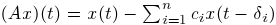and an application of the fixed-point index theorem, we obtain sufficient conditions for the existence, multiplicity and nonexistence of periodic solutions to a second-order differential equation with the prescribed neutral operator, which improve and extend some recent results of Lu-Ge, Wu-Wang, and Zhang. An example is given to illustrate our results. Moreover, the analysis of the generalized neutral operator will be helpful for other types of differential equations.

1: Paper Source PDF document

Paper's Title:

Approximation of Common Fixed Points of a Finite Family of Asymptotically Demicontractive Mappings in Banach Spaces

Author(s):

Yuchao Tang, Yong Cai, Liqun Hu and Liwei Liu

Department of Mathematics, NanChang University,
Nanchang 330031, P.R. China
Department of Mathematics, Xi'an Jiaotong University,
Xi'an 710049, P.R. China

hhaaoo1331@yahoo.com.cn

Abstract:

By virtue of new analytic techniques, we analyze and study
several strong convergence theorems for the approximation of
common fixed points of asymptotically demicontractive mappings
via the multistep iterative sequence with errors in Banach
spaces. Our results improve and extend the corresponding ones
announced by Osilike , Osilike and Aniagbosor, Igbokwe, Cho et
al., Moore and Nnoli, Hu and all the others.

1: Paper Source PDF document

Paper's Title:

Necessary and Sufficient Conditions for Cyclic Homogeneous Polynomial Inequalities of Degree Four in Real Variables

Author(s):

Vasile Cirtoaje and Yuanzhe Zhou

Department of Automatic Control and Computers
University of Ploiesti
Romania.
vcirtoaje@upg-ploiesti.ro.

High School Affiliated to Wuhan University, China

Abstract:

In this paper, we give two sets of necessary and sufficient conditions that the inequality f4(x,y,z) ≥ 0 holds for any real numbers x,y,z, where f4(x,y,z) is a cyclic homogeneous polynomial of degree four. In addition, all equality cases of this inequality are analysed. For the particular case in which f4(1,1,1)=0, we get the main result in . Several applications are given to show the effectiveness of the proposed methods.

1: Paper Source PDF document

Paper's Title:

On Convergence Theorems of an Implicit Iterative Process with Errors for a Finite Family of Asymptotically quasi I-nonexpansive Mappings

Author(s):

Farrukh Mukhamedov and Mansoor Saburov

Department of Computational & Theoretical Sciences,
Faculty of Sciences, International Islamic University Malaysia,
P.O. Box, 141, 25710, Kuantan,
Malaysia

farrukh_m@iiu.edu.my

msaburov@gmail.com

http://www.iium.edu.my

Abstract:

In this paper we prove the weak and strong convergence of the implicit iterative process with errors to a common fixed point of a finite family {Tj}Ni=1 of asymptotically quasi Ij-nonexpansive mappings as well as a family of {Ij}Nj=1 of asymptotically quasi nonexpansive mappings in the framework of Banach spaces. The obtained results improve and generalize the corresponding results in the existing literature.

1: Paper Source PDF document

Paper's Title:

Properties of Certain Multivalent Functions Involving Ruscheweyh Derivatives

Author(s):

N-Eng Xu and Ding-Gong Yang

Department of Mathematics,
Changshu Institute of Technology,
Changshu, Jiangsu 215500,
China

Abstract:

Let Ap(p∈ N) be the class of functions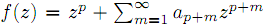which are analytic in the unit disk. By virtue of the Ruscheweyh derivatives we introduce the new subclasses Cp(n,α,β,λ,μ) of Ap. Subordination relations, inclusion relations, convolution properties and a sharp coefficient estimate are obtained. We also give a sufficient condition for a function to be in Cp(n,α,β,λ,μ)

1: Paper Source PDF document

Paper's Title:

Schwarz Method for Variational Inequalities Related to Ergodic Control Problems

Author(s):

Department of Mathematics, Badji Mokhtar University, Annaba 23000,
P.O.Box. 12, Annaba 23000, Algeria

signor_2000@yahoo.fr

Mcherihalima@yahoo.fr

Abstract:

In this paper, we study variational inequalities related to ergodic control problems studied by M. Boulbrachčne and H. Sissaoui , where the "discount factor" (i.e., the zero order term) is set to 0, we use an overlapping Schwarz method on nomatching grid which consists in decomposing the domain in two subdomains. For α ∈ ]0.1[ we provide the discretization on each subdomain converges in L -norm.

1: Paper Source PDF document

Paper's Title:

Stability of an Almost Surjective epsilon-Isometry in The Dual of Real Banach Spaces

Author(s):

Minanur Rohman, Ratno Bagus Edy Wibowo, Marjono

Department of Mathematics, Faculty of Mathematics and Natural Sciences,
Brawijaya University,
Jl. Veteran Malang 65145,
Indonesia.
E-mail: miminanira@gmail.com

Department of Mathematics, Faculty of Mathematics and Natural Sciences,
Brawijaya University,
Jl. Veteran Malang 65145,
Indonesia.
E-mail: rbagus@ub.ac.id

Department of Mathematics, Faculty of Mathematics and Natural Sciences,
Brawijaya University,
Jl. Veteran Malang 65145,
Indonesia.
E-mail: marjono@ub.ac.id

Abstract:

In this paper, we study the stability of epsilon-isometry in the dual of real Banach spaces. We prove that the almost surjective epsilon-isometry mapping is stable in dual of each spaces. The proof uses Gâteaux differentiability space (GDS), weak-star exposed points, norm-attaining operator, and some studies about epsilon-isometry that have been done before.

1: Paper Source PDF document

Paper's Title:

Hermite-Hadamard Type Inequalities for k-Riemann Liouville Fractional Integrals Via Two Kinds of Convexity

Author(s):

R. Hussain1, A. Ali2, G. Gulshan3, A. Latif4 and K. Rauf5

1,2,3,4Department of Mathematics,
Mirpur University of Science and Technology, Mirpur.
Pakistan.
E-mail1rashida12@gmail.com
E-mail2: unigraz2009@yahoo.com
E-mail3: ghazalagulshan@yahoo.com
E-mail4: asialatif87@gmail.com

5Department of Mathematics,
University of Ilorin, Ilorin,
Nigeria.
E-mail5: krauf@unilorin.edu.ng

Abstract:

In this article, a fundamental integral identity including the first order derivative of a given function via k-Riemann-Liouville fractional integral is established. This is used to obtain further Hermite-Hadamard type inequalities involving left-sided and right-sided k-Riemann-Liouville fractional integrals for m-convex and (s,m)-convex functions respectively.

1: Paper Source PDF document

Paper's Title:

Iterative Algorithm for Split Generalized Mixed Equilibrium Problem Involving Relaxed Monotone Mappings in Real Hilbert Spaces

Author(s):

1U.A. Osisiogu, F.L. Adum, and 2C. Izuchukwu

1Department of Mathematics and Computer Science,
Ebonyi State University, Abakaliki,
Nigeria.

2School of Mathematics, Statistics and Computer Science,
University of KwaZulu-Natal, Durban,
South Africa.
E-mail: izuchukwuc@ukzn.ac.za, izuchukwu_c@yahoo.com

Abstract:

The main purpose of this paper is to introduce a certain class of split generalized mixed equilibrium problem involving relaxed monotone mappings. To solve our proposed problem, we introduce an iterative algorithm and obtain its strong convergence to a solution of the split generalized mixed equilibrium problems in Hilbert spaces. As special cases of the proposed problem, we studied the proximal split feasibility problem and variational inclusion problem.

1: Paper Source PDF document

Paper's Title:

Convergence Speed of Some Random Implicit-Kirk-type Iterations for Contractive-type Random Operators

Author(s):

H. Akewe, K.S. Eke

Department of Mathematics,
Covenant University,
Canaanland, KM 10, Idiroko Road, P. M. B. 1023, Ota, Ogun State,
Nigeria.
E-mail: hudson.akewe@covenantuniversity.edu.ng, kanayo.eke@covenantuniversity.edu.ng

Abstract:

The main aim of this paper is to introduce a stochastic version of multistep type iterative scheme called a modified random implicit-Kirk multistep iterative scheme and prove strong convergence and stability results for a class of generalized contractive-type random operators. The rate of convergence of the random iterative schemes are also examined through an example. The results show that our new random implicit kirk multistep scheme perform better than other implicit iterative schemes in terms of convergence and thus have good potentials for further applications in equilibrium problems in computer science, physics and economics.

1: Paper Source PDF document

Paper's Title:

Some Inequalities of the Hermite-Hadamard Type for k-Fractional Conformable Integrals

Author(s):

C.-J. Huang, G. Rahman, K. S. Nisar, A. Ghaffar and F. Qi

Department of Mathematics, Ganzhou Teachers College,
Ganzhou 341000, Jiangxi,
China.
E-mail: hcj73jx@126.com , huangcj1973@qq.com

Department of Mathematics, Shaheed Benazir Bhutto University,
Sheringal, Upper Dir, Khyber Pakhtoonkhwa,
Pakistan.
E-mail: gauhar55uom@gmail.com

Department of Mathematics, College of Arts and Science at Wadi Aldawaser, 11991,
Prince Sattam Bin Abdulaziz University, Riyadh Region,
Kingdom of Saudi Arabia.
E-mail: n.sooppy@psau.edu.sa, ksnisar1@gmail.com

Department of Mathematical Science, Balochistan University of Information Technology,
Engineering and Management Sciences, Quetta,
Pakistan.
E-mail: abdulghaffar.jaffar@gmail.com

School of Mathematical Sciences, Tianjin Polytechnic University,
Tianjin 300387,
China; Institute of Mathematics,
Henan Polytechnic University, Jiaozuo 454010, Henan,
China.
E-mail: qifeng618@gmail.com, qifeng618@qq.com

Abstract:

In the paper, the authors deal with generalized k-fractional conformable integrals, establish some inequalities of the Hermite-Hadamard type for generalized k-fractional conformable integrals for convex functions, and generalize known inequalities of the Hermite-Hadamard type for conformable fractional integrals.

1: Paper Source PDF document

Paper's Title:

An Analytical Solution of Perturbed Fisher's Equation Using Homotopy Perturbation Method (HPM), Regular Perturbation Method (RPM) and Adomian Decomposition Method (ADM)

Author(s):

Moussa Bagayogo, Youssouf Minoungou, Youssouf Pare

Departement de Mathematique,
Universite Ouaga I Pr Joseph Ki-Zerbo,
Burkina Faso.
E-mail: moussabagayogo94@gmail.com, m.youl@yahoo.fr, pareyoussouf@yahoo.fr.

Abstract:

In this paper, Homotopy Perturbation Method (HPM), Regular Pertubation Method (RPM) and Adomian decomposition Method (ADM) are applied to Fisher equation. Then, the solution yielding the given initial conditions is gained. Finally, the solutions obtained by each method are compared

1: Paper Source PDF document

Paper's Title:

Dynamical Analysis of HIV/AIDS Epidemic Model with Two Latent Stages, Vertical Transmission and Treatment

Author(s):

Nur Shofianah, Isnani Darti, Syaiful Anam

Mathematics Department,Faculty of Mathematics and Natural Sciences.
University of Brawijaya,
Jl. Veteran, Malang 65145,
Indonesia.
E-mail: nur_shofianah@ub.ac.id, isnanidarti@ub.ac.id, syaiful@ub.ac.id

Abstract:

We discuss about dynamical analysis of HIV/AIDS epidemic model with two latent stages, vertical transmission and treatment. In this model, the spreading of HIV occurs through both horizontal and vertical transmission. There is also treatment for individual who has been HIV infected. The latent stage is divided into slow and fast latent stage based on the immune condition which varies for each individual. Dynamical analysis result shows that the model has two equilibrium points: the disease-free equilibrium point and the endemic equilibrium point. The existence and global stability of equilibrium points depend on the basic reproduction number R0. When R0 <1, only the disease-free equilibrium point exists. If R0 >1, there are two equilibrium points, which are the disease-free equilibrium point and the endemic equilibrium point. Based on the result of stability analysis, the disease-free equilibrium point is globally asymptotically stable if R0 <1, while if R0 > 1 and p=q, the endemic equilibrium point will be globally asymptotically stable. In the end, we show some numerical simulations to support the analytical result.

1: Paper Source PDF document

Paper's Title:

Some New Mappings Related to Weighted Mean Inequalities

Author(s):

Xiu-Fen Ma

College of Mathematical and Computer,
No.9 of Xuefu Road, Hechuan District 401520,
Chongqing City,
The People's Republic of China.
E-mail: maxiufen86@163.com

Abstract:

In this paper, we define four mappings related to weighted mean inequalities, investigate their properties, and obtain some new refinements of weighted mean inequalities.

1: Paper Source PDF document

Paper's Title:

Some fixed point results in partial S-metric spaces

Author(s):

M. M. Rezaee, S. Sedghi, A. Mukheimer, K. Abodayeh, and Z. D. Mitrovic

Department of Mathematics, Qaemshahr Branch,
Iran.

Department of Mathematics, Qaemshahr Branch,
Iran.
E-mail: sedghi.gh@qaemiau.ac.ir

Department of Mathematics and General Sciences,
KSA.
E-mail: mukheimer@psu.edu.sa

Department of Mathematics and General Sciences,
KSA.
E-mail: kamal@psu.edu.sa

Nonlinear Analysis Research Group,
Faculty of Mathematics and Statistics,
Ton Duc Thang University, Ho Chi Minh City,
Vietnam.
E-mail: zoran.mitrovic@tdtu.edu.vn

Abstract:

We introduce in this article a new class of generalized metric spaces, called partial S-metric spaces. In addition, we also give some interesting results on fixed points in the partial S-metric spaces and some applications.

1: Paper Source PDF document

Paper's Title:

Double Difference of Composition Operator on Bloch Spaces

Author(s):

Rinchen Tundup

Department of Mathematics
University of Jammu
Jammu and Kashmir
India.

E-mail: joneytun123@gmail.com

Abstract:

In this paper we characterize the compactness of double difference of three non-compact composition operators on Bloch space induced by three holomorphic self maps on the unit disc.

1: Paper Source PDF document

Paper's Title:

Solving Non-Autonomous Nonlinear Systems of Ordinary Differential Equations Using Multi-Stage Differential Transform Method

Author(s):

K. A. Ahmad, Z. Zainuddin, F. A. Abdullah

School of Mathematical Sciences Universiti Sains Malaysia
11800 USM Penang
Malaysia.
zarita@usm.my
farahaini@usm.my

Abstract:

Differential equations are basic tools to describe a wide variety of phenomena in nature such as, electrostatics, physics, chemistry, economics, etc. In this paper, a technique is developed to solve nonlinear and linear systems of ordinary differential equations based on the standard Differential Transform Method (DTM) and Multi-stage Differential Transform Method (MsDTM). Comparative numerical results that we are obtained by MsDTM and Runge-Kutta method are proposed. The numerical results showed that the MsDTM gives more accurate approximation as compared to the Runge-Kutta numerical method for the solutions of nonlinear and linear systems of ordinary differential equations

1: Paper Source PDF document

Paper's Title:

Fractional exp(-φ(ξ))- Expansion Method and its Application to Space--Time Nonlinear Fractional Equations

Author(s):

A. A. Moussa and L. A. Alhakim

Department of Management Information System and Production Management,
College of Business and Economics, Qassim University,
P.O. BOX 6666, Buraidah: 51452,
Saudi Arabia.
E-mail: Alaamath81@gmail.com

Department of Management Information System and Production Management,
College of Business and Economics, Qassim University,
P.O. BOX 6666, Buraidah: 51452,
Saudi Arabia.
E-mail: Lama2736@gmail.com

Abstract:

In this paper, we mainly suggest a new method that depends on the fractional derivative proposed by Katugampola for solving nonlinear fractional partial differential equations. Using this method, we obtained numerous useful and surprising solutions for the space--time fractional nonlinear Whitham--Broer--Kaup equations and space--time fractional generalized nonlinear Hirota--Satsuma coupled KdV equations. The solutions obtained varied between hyperbolic, trigonometric, and rational functions, and we hope those interested in the real-life applications of the previous two equations will find this approach useful.

1: Paper Source PDF document

Paper's Title:

Coexisting Attractors and Bubbling Route to Chaos in Modified Coupled Duffing Oscillators

Author(s):

B. Deruni1, A. S. Hacinliyan1,2, E. Kandiran3, A. C. Keles2, S. Kaouache4, M.-S. Abdelouahab4, N.-E. Hamri4

1Department of Physics,
University of Yeditepe,
Turkey.

2Department of Information Systems and Technologies,
University of Yeditepe,
Turkey

3Department of Software Development,
University of Yeditepe,
Turkey.

4Laboratory of Mathematics and their interactions,
University Center of Abdelhafid Boussouf,
Mila 43000,
Algeria.

E-mail: berc890@gmail.com
ahacinliyan@yeditepe.edu.tr
engin.kandiran@yeditepe.edu.tr
cihan.keles@yeditepe.edu.tr
s.kaouache@centr-univ-mila.dz
medsalah3@yahoo.fr
n.hamri@centre-univ-mila.dz

Abstract:

In this article dynamical behavior of coupled Duffing oscillators is analyzed under a small modification. The oscillators have cubic damping instead of linear one. Although single duffing oscillator has complex dynamics, coupled duffing systems possess a much more complex structure. The dynamical behavior of the system is investigated both numerically and analytically. Numerical results indicate that the system has double scroll attractor with suitable parameter values. On the other hand, bifurcation diagrams illustrate rich behavior of the system, and it is seen that, system enters into chaos with different routes. Beside classical bifurcations, bubbling route to chaos is observed for suitable parameter settings. On the other hand, Multistability of the system is indicated with the coexisting attractors, such that under same parameter setting the system shows different periodic and chaotic attractors. Moreover, chaotic synchronization of coupled oscillators is illustrated in final section.

1: Paper Source PDF document

Paper's Title:

On Euler's First Transformation Formula for k-hypergeometric Function

Author(s):

Sungtae Jun and Insuk Kim

General Education Institute,
Konkuk University, Chungju 380-701,
Republic of Korea.
E-mail: sjun@kku.ac.kr

Department of Mathematics Education,
Wonkwang University, Iksan, 570-749,
Republic of Korea.
E-mail: iki@wku.ac.kr

Abstract:

Mubeen et al. obtained Kummer's first transformation for the k-hypergeometric function. The aim of this note is to provide the Euler-type first transformation for the k-hypergeometric function. As a limiting case, we recover the results of Mubeen et al. In addition to this, an alternate and easy derivation of Kummer's first transformation for the k-hypergeometric function is also given.

Search and serve lasted 1 second(s).